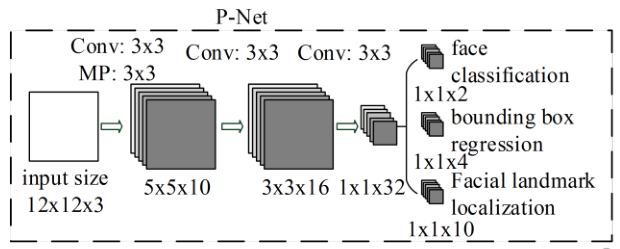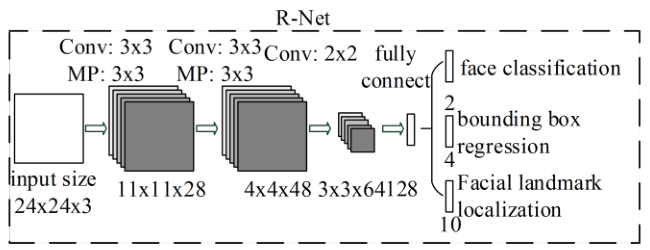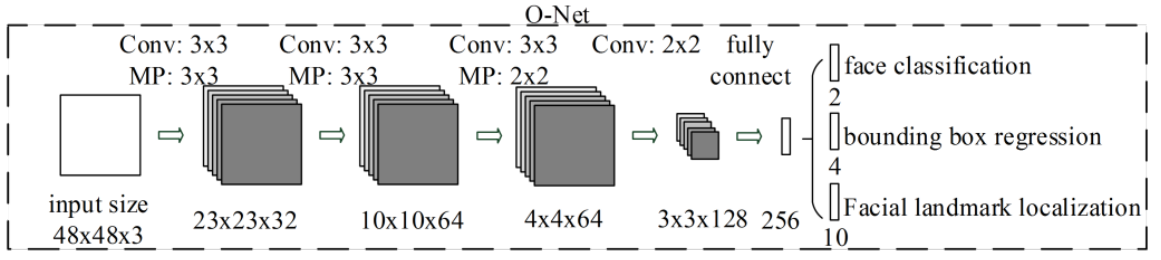@ArrowLLL 2018-07-27T12:23:31.000000Z 字数 4327 阅读 1102

# Study-Note: Joint Face Detection and Alignment using Multi-task Cascaded Convolutional Networks

Study-Note face-recognization

problem: Face detection and alignment

This paper proposes a new framework to integrate these two tasks using unified cascaded CNNs by multi-task learning. The proposed CNNs consist of three stages:

1. produces candidate windows quickly through a shallow CNN
2. refines the windows to reject a large number of non-faces windows through a more complex CNN
3. use a more powerful CNN to refine the result and output facial landmarks positions.

Contribution
1. propose a new cascaded CNNs based frame work for joint face detection and alignment, and carefully design lightweight CNN architrcture for real time performance.
2. propose an effective method to conduct online hard sample mining to improve the performance.
3. Extensive experiments are conducted on challenging benchmarks

## Approach

Given an image, we initially resize it to different scales to build an image pyramid, which is the input of the following three-stage cascaded framework.

### Stage 1: P-Net(Proposal Network)1. use manner in 《Multi-view face detection using deep convolutional neural networks》to obtain the candidate windows and their bounding box regressionvectors;

### Stage 2: R-Net(Refine Net)• input all candidates outputed from P-Net to reject a large number of false candidates;
• perform calibration with bounding box regression and NMS candidate merge;

### Stage 3: O-Net(Output Network)aim to describe the face in more details, output five facial landmarks' position

## Training

leverage three tasks to train our CNN detectors, which were output of three stages' output.

• face/non-face classification

for each sample $x_i$, we use the cross-entropy loss:

• $p_i$ is the probality pruduced by the network that indicates a sample being a face.
• $y_i^{det} \in \{0, 1\}$ denotes the ground-truth label
• bounding box regression

Predict the offset between each candidate window and the nearest ground truth, we employ the Euclidean loss for each sample $x_i$

• $\hat{y}_i^{box}$ regression target obtained from the network
• $y_i^{box}$ is the ground-truth coordinate.
• $\hat{y}_i^{box}, y_i^{box} \in \mathbb{R}^4$, including [left, top, height, width]
• facial landmark localization

also be formulated as a regression problem and we minimize the Euclidean loss:

• $\hat{y}_i^{landmark}$ is the facial landmark's coordinate obtained from the network
• $y_i^{landmark}$ is the groundtruth coordinate
• $\hat{y}_i^{landmark}, y_i^{landmark} \in \mathbb{R}^{10}$, including [L_eye, R_eye, nose, left mouth corner, right mouth corner], $(x, y)$ for every element.
• Multi-source training

The overall learning target can be formulated as below to synthesize three different tasks

• $N$ is the number of training sapmle
• $\alpha_j$ denotes on the task importance
• $\alpha_{det} = 1, \alpha_{box} = 0.5, \alpha_{landmark} = 0.5$ in P-Net and R-Net
• $\alpha_{det} = 1, \alpha_{box} = 0.5, \alpha_{landmark} = 1$ in O-Net
• $\beta_i^j \in \{0, 1\}$ is the sample type indicator. for different types of training images in the learning process, such as face, non-face and partially aligned face.
• use non-face as an example, we only compute $L_i^{det}$, so the other are set to $0$. This is the meaning of $\beta$ as a sample type indicator.
• Online Hard sample mining mining

in each mini-batch, we sort the loss computed in the forward propagation phase from all samples and select the top 70% of them as hard samples. Then we only compute the gradient from the hard samples in the backward propagation phase. That means we ignore the easy samples that are less helpful to strengthen the detector while training.

## Experiments

### Annitation

Four different kinds of data annotation:

1. Negatives: Regions that the Intersection-over-Union(IoU) ratio less than 0.3 to any ground-truth;
2. Positive: IoU above 0.65 to a ground truth;
3. Part faces: IoU between 0.4 and 0.65 to a ground-truth;
4. Landmark faces: faces labeled 5 landmarks' positions

and

• Negatives and positivesare used for face classification tasks;
• positives and part faces are used for bounding box regression;
• landmark faces are used for facial landmark localization

### training data

WIDER FACE and CelebA

## 拓展阅读

stage_1的模型基础: Multi-view Face Detection Using Deep Convolutional Neural Networks
multiple CNNs for face detection: A Convolutional Neural Network Cascade for Face Detection
Online Hard sample mining: Training Region-Based Object Detectors With Online Hard Example Mining
landmark 比较对象：Facial Landmark Detection by Deep Multi-task Learning• 私有
• 公开
• 删除